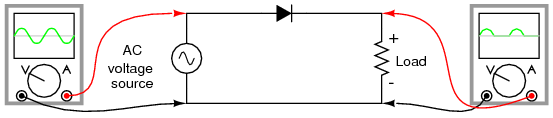This is the electricity part of the Literature Review moved from HSU Chiapas Windbelt ASE.

### Electricity

• A rectifier is a device that has a high resistance of current flow in one direction, but not in the other. It is commonly used to convert alternating current to direct current. It will be necessary for our design team to build a rectifier in order to obtain accurate readings.

#### Series and Parallel Circuits

These two video lectures help explain how series and parallel circuits are calculated, measured, and overall understood.

#### Diodes and Capacitors

This book talks about electricity basics. The part that is particularly useful for our group are the parts about diodes and capacitors. Diodes convert AC current into DC current. Capacitors can do many things, for example, they can store electrical energy to use later, stop DC current from going through circuits, smooth out voltage, make a timer to monitor energy levels, and filtering signals/frequencies (like in radios).

#### Electromagnetic Force and Electromagnetic Induction

• Michael Faraday discovered electromagnetic induction in 1831, he hypothesized that a changing magnetic field is necessary to induce a current in a nearby circuit. To test this he made a coil by wrapping a paper cylinder with wire. He connected the coil to a galvanometer, and then moved a magnet back and forth inside the cylinder.
• When Michael Faraday made his discovery of electromagnetic induction in 1831, he hypothesized that a changing magnetic field is necessary to induce a current in a nearby circuit. To test his hypothesis he made a coil by wrapping a paper cylinder with wire. He connected the coil to a galvanometer, and then moved a magnet back and forth inside the cylinder.
• When he move the magnet back and forth inside the cylinder he notice that the galvanometer needle moves, indicating that a current is induced in the coil. He also noticed that the needle immediately returns to zero when the magnet is not moving. Faraday confirmed that a moving magnetic field is necessary in order for electromagnetic induction to occur.

• Faraday found that the electromotive force (EMF) produced around a closed path is proportional to the rate of change of the magnetic flux through any surface bounded by that path.
• In other words, an electrical current is induced to any closed circuit when the magnetic flux changes. This happens when the magnetic field itself changes or when the conductor, or magnet, is moved through the circuit.
• Electromagnetic induction underlies the operation of generators, all electric motors, transformers, induction motors, synchronous motors, solenoids, and most other electrical machines.
• Faraday's law of electromagnetic induction states that:
   \mathcal{E} = -{{d?B} \over dt},


Thus:

   \mathcal{E} is the electromotive force (emf) in volts
?B is the magnetic flux in webers(In physics, a weber is the SI unit of magnetic flux. A "flux density" of one Wb/m2, or one weber per square meter is one tesla. The weber is named for the German physicist Wilhelm Eduard Weber 1804-1891.)

• For the common but special case of a coil of wire, composed of N loops with the same area, Faraday's law of electromagnetic induction states that
   \mathcal{E} = - N{{d?B} \over dt}


where

   \mathcal{E} is the electromotive force (emf) in volts
N is the number of turns of wire
?B is the magnetic flux in webers through a single loop.


• [from journal]
• This article explains electromagnetic force in a simple manner. It talks about a flash light called the "No Batteries Light" that needs no batteries to operate and emits light due to an electromagnetic force produced as the item is shaken or moved. Moving or shaking the flashlight passes a magnet in and out through a coil, producing energy. In other words, the introduction of a magnetic field into an electric field, produces electrical current.
•This link is helpful, simple information about how rectifier circuits work, plus there are various images to aid in understanding the process.